# Dividing Fractions, CCSS.Math.Content.5.NF.B.7

##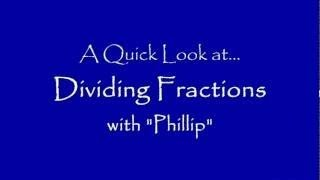By mathtrain

6th Grader at Lincoln Middle School in Santa Monica, CA shows us how to divide fractions. (We use a Tablet PC and Camtasia Studio.) See Mathtrain.TV or our iTunes Podcast. CCSS.Math.Content.5.NF.B.7 .# Grade 5 Math - Divide Decimals

##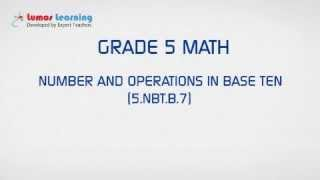By Lumos Learning

Using the Lumos Study Programs, parents and educators can reinforce the classroom learning experience for children and help them succeed at school and on the standardized tests. Lumos books, dvd, eLearning and tutoring are used by leading schools, libraries and thousands of parents to supplement classroom learning and improve student achievement in the standardized tests.# Grade 5 Math - Dividing Fractions

##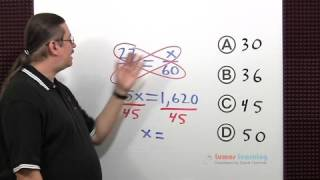By Lumos Learning

Using the Lumos Study Programs, parents and educators can reinforce the classroom learning experience for children and help them succeed at school and on the standardized tests. Lumos books, dvd, eLearning and tutoring are used by leading schools, libraries and thousands of parents to supplement classroom learning and improve student achievement in the standardized tests.# Dividing whole numbers by unit fractions

##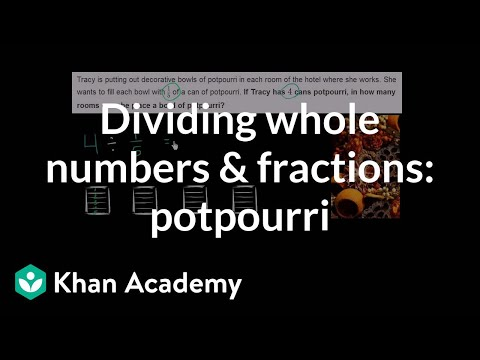By Khan Academy

Solve a word problem by dividing a whole number by a fraction. The example used in this video is 4 ï¿½ï¿½ï¿½ï¿½ï¿½ï¿½ï¿½ï¿½ï¿½ï¿½ï¿½ï¿½ 1/5.# Grade 5 Math - Dividing Fractions 1

##By Lumos Learning

Using the Lumos Study Programs, parents and educators can reinforce the classroom learning experience for children and help them succeed at school and on the standardized tests. Lumos books, dvd, eLearning and tutoring are used by leading schools, libraries and thousands of parents to supplement classroom learning and improve student achievement in the standardized tests.

PARENTS please visit LumosTestPrep.com to learn more.
EDUCATORS please visit LumosLearning.com to learn more# Dividing unit fractions by whole numbers

##By Khan Academy

Solve a word problem by dividing a fraction by a whole number. The example used in this video is 1/5 ï¿½ï¿½ï¿½ï¿½ï¿½ï¿½ï¿½ï¿½ï¿½ï¿½ï¿½ï¿½ 4.# eSpark Learning: Dividing Fractions Instructional Video (5.NF.3)

##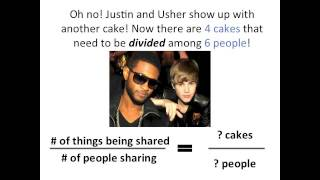By eSparkLearningVideos

This eSpark Learning instructional video aligns with Common Core State Standard 5.NF.3: interpret a fraction as division of the numerator by the denominator. Solve word problems involving division of whole numbers leading to answers in the form of fractions or mixed numbers.# [5.NBT.2-2.0] Multiplying/Dividing by 10 - Common Core Standard

##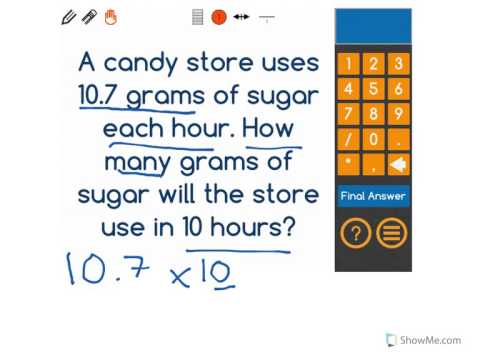By Front Row

Discover more Common Core Math at https://www.frontrowed.com Explain patterns in the placement of the decimal point when a decimal is multiplied by a power of 10, use whole-number exponents to denote powers of 10 Front Row is a free, adaptive, Common Core aligned math program for teachers and students in kindergarten through eighth grade. Front Row allows students to practice math at their own pace - learning advanced concepts when they're ready and receiving remediation when they struggle. Front Row provides teachers with access to a detailed data dashboard and weekly email reports that show which standards are causing students difficulty, what small groups can be formed for interventions, and how their students are progressing in math.Discover more Common Core Math at https://www.frontrowed.com# [5.NBT.2-3.0] Multiplying/Dividing by 10 - Common Core Standard

##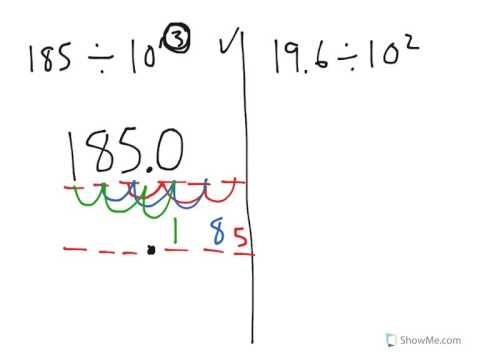By Front Row

Discover more Common Core Math at https://www.frontrowed.comExplain patterns in the placement of the decimal point when a decimal is divided by a power of 10, use whole-number exponents to denote powers of 10Front Row is a free, adaptive, Common Core aligned math program for teachers and students in kindergarten through eighth grade. Front Row allows students to practice math at their own pace - learning advanced concepts when they're ready and receiving remediation when they struggle. Front Row provides teachers with access to a detailed data dashboard and weekly email reports that show which standards are causing students difficulty, what small groups can be formed for interventions, and how their students are progressing in math.Discover more Common Core Math at https://www.frontrowed.com# Dividing Polynomials

##By Carter Maslan

step-by-step example of dividing the polynomial (x^2 + 3x - 40) / (x - 5).# DEPRECATED Multiplying and dividing rational expressions 5

##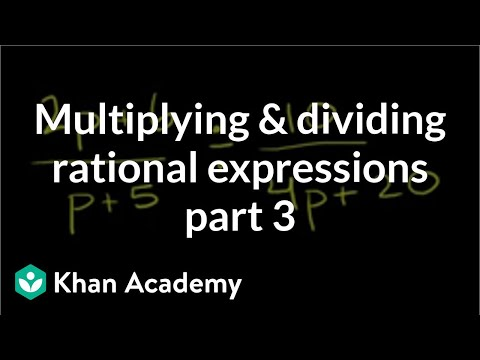By Khan Academy

Sal performs the following division and simplifies the result: (2p+6)/(p+5) ï¿½ï¿½ï¿½ï¿½ï¿½ï¿½ï¿½ï¿½ï¿½ï¿½ï¿½ï¿½ï¿½ï¿½ï¿½ï¿½ï¿½ï¿½ï¿½ï¿½ï¿½ (10)/(4p+20).# DEPRECATED Multiplying and dividing rational expressions 5

##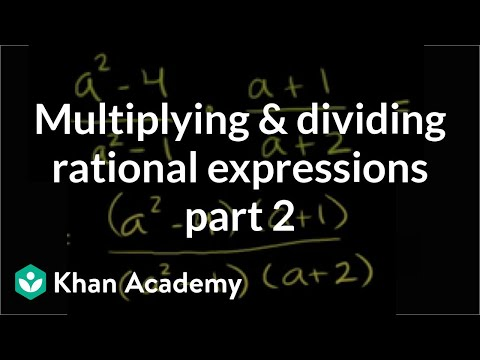By Khan Academy

Sal performs the following multiplication and simplifies the result: (a^2-4)/(a^2-1) X (a+1)/(a+2).# 5.NF.B.7C.a. Fraction Divided by Whole Number

##By LANL MSA# Multiplication and Division Fact Families

##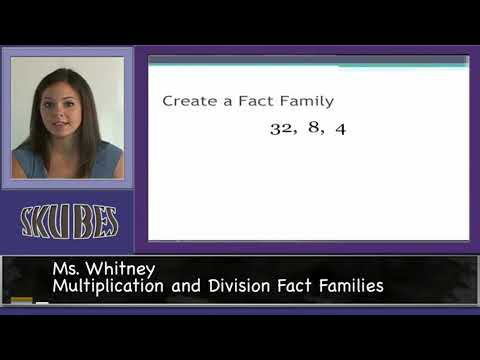By Skubes ed

3.OA.7 Fluently multiply and divide within 100, using strategies such as the relationship between multiplication and division (e.g., knowing that 8 × 5 = 40, one knows 40 ÷ 5 = 8) or properties# [5.NBT.2-1.0] Multiplying/Dividing by 10 - Common Core Standard - Practice Problem

##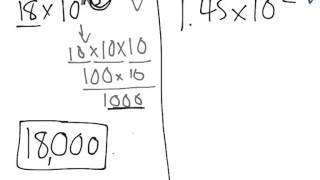By Front Row

Explain patterns in the number of zeros of the product when multiplying a number by powers of 10, use whole-number exponents to denote powers of 10# eSpark Learning: Dividing Fractions Instructional Video (5.NF.3)

##By eSparkLearningVideos# Dividing whole numbers by unit fractions

##By Khan Academy

Solve a word problem by dividing a whole number by a fraction. The example used in this video is 4 ï¿½ï¿½ï¿½ï¿½ï¿½ï¿½ï¿½ï¿½ï¿½ï¿½ï¿½ï¿½ 1/5.# Dividing whole numbers by unit fractions

##By Khan Academy

Solve a word problem by dividing a whole number by a fraction. The example used in this video is 4 ï¿½ï¿½ï¿½ï¿½ï¿½ï¿½ï¿½ï¿½ï¿½ï¿½ï¿½ï¿½ 1/5.# Dividing unit fractions by whole numbers

##By Khan Academy

Solve a word problem by dividing a fraction by a whole number. The example used in this video is 1/5 ï¿½ï¿½ï¿½ï¿½ï¿½ï¿½ï¿½ï¿½ï¿½ï¿½ï¿½ï¿½ 4.# Dividing unit fractions by whole numbers

##By Khan Academy

Solve a word problem by dividing a fraction by a whole number. The example used in this video is 1/5 ï¿½ï¿½ï¿½ï¿½ï¿½ï¿½ï¿½ï¿½ï¿½ï¿½ï¿½ï¿½ 4.